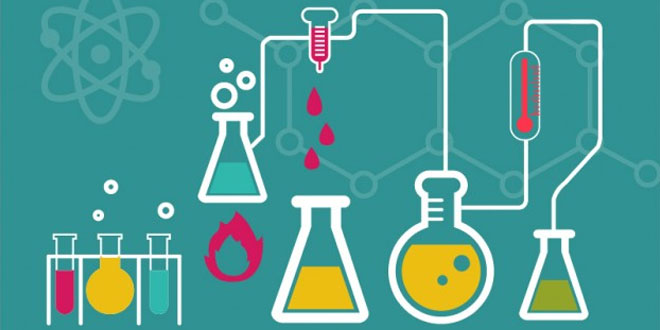Friday , August 14 2020# 10th CBSE Science Annual Exam 2017-18

Time: 3 hours
M.M.: 80
Class: 10th
Subject: Science

## General Instructions:

• The Question paper comprises two Section – A and B. You are to attempt both the sections.
• All questions are compulsory.
• All questions of Section A and Section B are to be attempted separately.
• There is an internal choice in three questions of three marks each, two questions of five marks each in Section A and one question of two marks in Section B.
• Question numbers 1 and 2 in Section A are one mark questions. They are to be answered in one words or in one sentence.
• Question numbers 3 to 5 in Section A are two marks questions. These are to be answered in about 30 words each.
• Question numbers 6 to 15 in Section A are three marks questions. These are to be answered in about 50 words each.
• Question numbers 16 to 21 in Section A are five marks questions. These are to be answered in about 70 words each.
• Question numbers 22 to 27 in Section B are based on practical skills. Each question is a two marks question. These are to be answered in brief.

## Section A

#### Question: 4.

1. Name one gustatory receptor and one olfactory receptor present in human beings.
2. Write a and b in the given flow chart of neuron through which information travels as an electrical impulse.
Dendrite  →  a  →  b  →  End point of Neuron.

#### Question: 8.

1. Why are most carbon compounds poor conductors of electricity?
2. Write the name and structure of a saturated compound in which the carbon atoms are arranged in a ring. Give the number of single bonds present in this compound.

#### Or

1. Write Joule’s law of heating.
2. Two lamps, one rated 100 W; 220 V, and the other 60 W; 220 V, are connected in parallel to electric mains supply. Find the current drawn by two bulbs from the line, if the supply voltage is 220 V.

#### Question: 14. Students in a school listened to the news read in the morning assembly that the mountain of garbage in Delhi, suddenly exploded and various vehicles got buried under it. Several people were also injured and there was traffic jam all around. In the brain storming session the teacher also discussed this issue and asked the students to find out a solution to the problem of garbage. Finally they arrived at two main points – one is self management of the garbage we produced and the second is to generate less garbage at individual level.

1. Suggest two measures to manage the garbage we produce.
2. As an individual, what can we do to generate the least garbage? Give two points.
3. List two values the teacher instilled in his students in this episode.

#### Question: 16.

1. Write the steps involved in the extraction of pure metals in the middle of the activity series from their carbonate ores.
2. How is copper extracted from its sulphide one? Explain the various steps supported by chemical equations. Draw labelled diagram for the electrolytic refining of copper.

#### Question: 17.

1. The modern period table has been evolved through the early attempts of Dobereiner, Newland and Mendeleev. List one advantage and one limitation of all the three attempts.
2. Name the scientist who first of all showed that atomic number of an elements is more fundamental property than is atomic mass.
3. State Modern periodic law.

#### Question: 18.

1. Mention any two components of blood.
2. Trace the movement of oxygenated blood in the body.
3. Write the function of valved present in between atria and ventricles.
4. Write one structural difference between the composition of artery and veins.

#### Or

1. Define excretion.
2. Name the basic filtration unit present in the kidney.
3. Draw excretory system in human beings and label the following organs of excretory system which perform following functions:
(i) form urine.
(ii) is a long tube which collects urine from kidney.
(iii) store urine until it is passed out.

#### Question: 19.

1. Write the function of following parts in human female reproductive system:
(i) Ovary (ii) Oviduct (iii) Uterus
2. Describe in brief the structure and function of placenta.

#### Or

1. Write the function of each of the following parts of human eye:
(i) Cornea                       (ii) Iris
(iii) Crystalline lens   (iv) Ciliary muscle
2. Why does the sun appear reddish early in the morning? Will this phenomenon be observed by an astronaut on the Moon? Give reason to justify your answer.

#### Question: 21.

1. State Fleming’s left hand rule.
2. Write the principle of working of an electric motor.
3. Explain the function of the following parts of an electric motor:
(i) Armature
(ii) Brushes
(iii) Split ring

## Light: 7th Class NCERT CBSE Science Chapter 15

Light 7th Class NCERT CBSE Science Chapter 15 Question: Briefly mention whether mirror change the direction of …